# Time + acceleration - examples

1. GravitationFrom the top of the 80m high tower, the body is thrown horizontally with an initial speed of 15 m/s. At what time and at what distance from the foot of the tower does the body hit the horizontal surface of the Earth? (use g = 10 ms-2)
2. Rocket start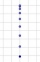The body launched vertically up returns to the start site in 6 seconds. What height did it have?
3. Reconstruction of the corridorCalculate how many minutes will be reduced to travel 187 km long railway corridor, where the maximum speed increases from 120 km/h to 160 km/h. Calculate how many minutes will shorten travel time, if we consider that the train must stop at 6 stations, eac
4. Free fall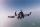Lloyd fall from height 7 m. Calculate the speed he hit the ground when falling with acceleration g = 9.81 m/s2
5. Fall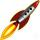The body was thrown vertically upward at speed v0 = 79 m/s. Body height versus time describe equation ?. What is the maximum height body reach?
6. Circular motionMass point moves moves uniformly in a circle with radius r = 3.4 m angular velocity ω = 3.6 rad/s. Calculate the period, frequency, and the centripetal acceleration of this movement.
7. Subway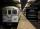Subway train went between two stations that gradually accelerated for 26 seconds and reached a speed of 72 km/h. At this rate, went 56 seconds. Then 16 seconds slowed to a stop. What was the distance between the stations?
8. AbyssStone was pushed into the abyss: 2 seconds after we heard hitting the bottom. How deep is the abyss (neglecting air resistance)? (gravitational acceleration g = 9.81 m/s2 and the speed of sound in air v = 343 m/s)
9. Train 2The train slowed down from 90 km/h to 72 km/h in 5 seconds. How long track travel?
10. BomberThe aircraft flies at an altitude of 4100 m above the ground at speed 777 km/h. At what horizontal distance from the point B should be release any body from the aircraft body to fall into point B? (g = 9.81 m/s2)
11. AccelerationThe car accelerates at rate 0.5m/s2. How long travels 400 meters and what will be its speed?
12. Traffic collisionWhen investigating a traffic accident, it was found that the driver stopped the vehicle immediately after the accident by constant braking on a 150 m track in 15 seconds. Do you approve that the driver exceeded the permitted speed (50 km/h) in the village.
13. FlywheelFlywheel turns 450 rev/min (RPM). Determine the magnitude of the normal acceleration of the flywheel point which are at a distance of 10 cm from the rotation axis.
14. G forcesCalculate deceleration of car (as multiple of gravitational acceleration g = 9.81 m/s2) which occurs when a car in a frontal collision slows down uniformly from a speed 111 km/h to 0 km/h in 1.2 meters trajectory.
15. Acceleration 2if a car traveling at a velocity of 80 m/s/south accelerated to a velocity of 100 m/s east in 5 seconds, what is the cars acceleration? using Pythagorean theorem
16. The tram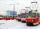The tram is moving with acceleration a = 0.3m/s2. How long it will pass the first meter of track? How long does it take 10 meters. What is its speed at the end of the 10 meters track?
17. Free fall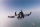How long does the stone fall freely into a depth of 80m? What speed will it hit the bottom of the abyss?
18. Orlík hydroelectric plantThe Orlík hydroelectric power plant, built in 1954-1961, consists of four Kaplan turbines. For each of them, the water with a flow rate of Q = 150 m3/s is supplied with a flow rate of h = 70.5 m at full power. a) What is the total installed power of the p
19. OverloadWhat overload in g (g-force) has passed the pilot if he accelerated from 0 to 600 km/h in 3 seconds?
20. DriverThe driver of the car at a speed of 100 km/h faced the obstacle and began to brake with a slowing of 5 m/s². What is the path to stopping the car when the driver has registered the obstacle with a delay of 0.7 s?

Do you have an interesting mathematical example that you can't solve it? Enter it, and we can try to solve it.

To this e-mail address, we will reply solution; solved examples are also published here. Please enter e-mail correctly and check whether you don't have a full mailbox.